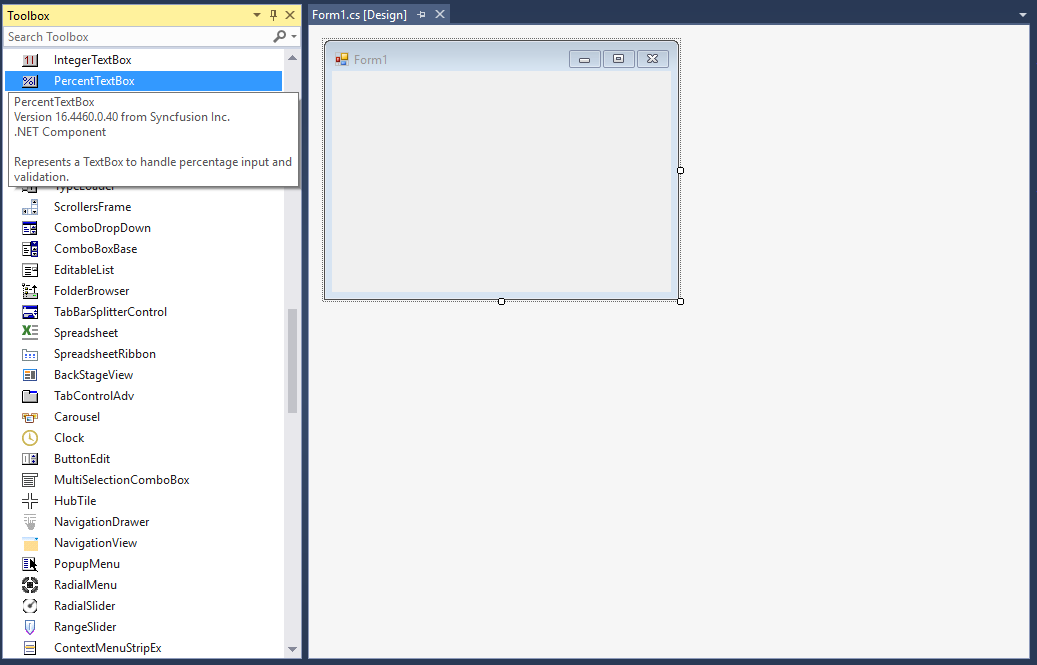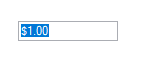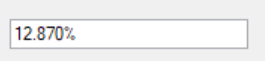# Getting Started with Windows Forms Percent TextBox

## Assembly deployment

Refer to the control dependencies section to get the list of assemblies or NuGet package that needs to be added as a reference to use the control in any application.

You can find more details about installing the NuGet packages in a Windows Forms application in the following link:

How to install nuget packages

### Create a simple application with PercentTextBox

You can create a Windows Forms application with PercentTextBox using the following steps:

### Create a project

Create a new Windows Forms project in Visual Studio to display the PercentTextBox control.

The PercentTextBox control can be added to an application by dragging it from the toolbox to a designer view. The Syncfusion.Shared.Base assembly reference will be added automatically:## Add control manually in code

1. Add the Syncfusion.Shared.Base assembly reference to the project:

2. Include the Syncfusion.Windows.Forms.Tools namespace.

``using Syncfusion.Windows.Forms.Tools;``
``Imports Syncfusion.Windows.Forms.Tools``

3. Create a PercentTextBox instance, and add it to the window.

``````PercentTextBox currencyTextBox1= new PercentTextBox();
``````Dim currencyTextBox1 As PercentTextBox = New PercentTextBox()## Maximum and minimum value constraints

You can set the maximum and minimum percentage values using the MaxValue and MinValue properties of PercentTextBox.

``````this.percentTextBox1.MaxValue = 6;
this.percentTextBox1.MinValue = -6;``````
``````Me.percentTextBox1.MaxValue = 6
Me.percentTextBox1.MinValue = -6``````

## Change number format

You can customize the number format using the PercentDecimalDigits, PercentDecimalSeparator, PercentGroupSeparator, and PercentGroupSizes properties of PercentTextBox.

``````this.percentTextBox1.PercentValue = 12.873;
this.percentTextBox1.PercentDecimalDigits = 3;
this.percentTextBox1.PercentDecimalSeparator = ".";
this.percentTextBox1.PercentGroupSeparator = ",";
this.percentTextBox1.PercentGroupSizes = new int[] { 2 };``````
``````Me.percentTextBox1.PercentValue = 12.873
Me.percentTextBox1.PercentDecimalDigits = 3
Me.percentTextBox1.PercentDecimalSeparator = "."
Me.percentTextBox1.PercentGroupSeparator = ","
Me.percentTextBox1.PercentGroupSizes = new int[] { 2 }``````